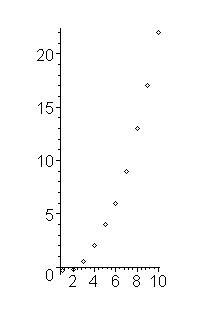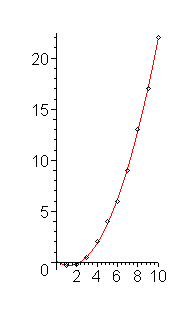# Introduction

The previous topic examined the most common form of linear regression, that of finding the best fitting straight line to data which is known to be linear in behaviour.

This next topic shows how we can find an arbitrary linear combination of basis functions of the form

y(x) = c1 f1(x) + c2 f2(x) + ⋅⋅⋅ + cn fn(x)

which best fits a given set of data. ≤

This technique is a straight-forward generalization of the Vandermonde method.

# General Linear Regression

Just like we may generalize interpolation to use a linear combination of basis functions, we may also do so with least squares. The technique is identical to the generalization of finding an interpolating straight line passing through two points to the finding of the best fitting least-squares line which passes through n points.

If we are trying to fit the linear combination of m basis functions:

y(x) = c1 f1(x) + c2 f2(x) + ⋅ ⋅ ⋅ + cm fm(x)

to a set of points, we define the generalized Vandermonde matrix V where each column are the x values evaluated at the jth basis function (j = 1, 2, ..., m), and then we solve:

VTVc = VTy

This gives us the vector of coefficients which give us the best fitting curve.

# Problem

Given data (xi, yi), for i = 1, 2, ..., n which is known to approximate a curve described by the following linear combination of basis functions:

y(x) = c1 f1(x) + c2 f2(x) + ⋅ ⋅ ⋅ + cm fm(x)

find the coefficients which define that best fitting curve.

# Assumptions

We will assume the model is correct and that the data is defined by two vectors x = (xi) and y = (yi). Additionally, we must assume that the number of unique values of x is at least as great as m and that the m basis functions are linearly independent.

# Tools

We will use linear algebra.

# Process

We wish to find the best fitting line of the form given form, we define the following generalized Vandermonde matrix:

where the first column is the function f1(x) evaluated at each of the x values, the second column is the function f2(x) evaluated at each of the x values, and so on.

Hence, we solve the linear system VTVc = VTy.

Having found the coefficient vector c, we now associate the appropriate entries with the appropriate basis function: y(x) = c1 f1(x) + c2 f2(x) + ⋅ ⋅ ⋅ + cm fm(x)

# Error Analysis

The study of the error associated with a linear regression is beyond the scope of this class. Se any text on linear regression, such as Draper and Smith, Applied Regression Analysis, 2nd Ed.

# Example 1

The following data is known to be quadratic in nature:

(1, -0.3), (2, -0.2), (3, 0.5), (4, 2), (5, 4),
(6, 6), (7, 9), (8, 13), (9, 17), (10, 22)

This data is shown in figure 1.Figure 1. The given data points.

Finding the technique of least squares, we define:

We now solve VTVc = VTy to get c = (0.2928, -0.75659, 0.18833)T.

Therefore, the best fitting quadratic curve using the least-squares technique is y(x) = 0.29280x2 - 0.75659x + 0.18833, which is shown in Figure 2.Figure 2. The best-fitting quadratic function using least squares.

# Questions

1. Find the least-squares quadratic polynomial which fits the data:

x = (-2, -1, 0, 1, 2)T
y = (3, 1, 0, 1, 5)T

Answer: y = x2 + 0.4 x + 0.

2. Find the least-squares curve of the form y = asin(0.4x) + bcos(0.4x) for the data

```>> x = (0:10)';
>> y = [2.29 1.89 1.09 0.230 -0.801 -1.56 -2.18 -2.45 -2.29 -1.75 -1.01]';
```

3. Given the same data in Question 2, find the best fitting curve of the form y = asin(0.4x) + bcos(0.4x) + c. Would you consider the constant coefficient to be significant? (While this is posed as a thought-provoking question here, there are statistical techniques to determine if a particular coefficient is significant: determine the standard deviation of each parameter and see if 0 falls within 1.96 standard deviations of the coefficient.)

Answer: 2.318cos(0.4x) − 0.6860sin(0.4x) − 0.006986.

# Applications to Engineering

Behaviour in engineering is most often linear or quadratic in behaviour. In these cases, linear regression to lines of the form:

• y(x) = c1 x + c2
• y(x) = c1 x2 + c2 x + c3

may be appropriate. However, in certain cases, additional information may be available, for example, the linear data may be known to pass through the origin (as is the case when considering the voltage across a resistor when the current is 0) or the voltage caused by the angle of a joystick when it is known that when the joystick is upright, the voltage is zero. In these two cases, it would be more appropriate to choose the following curves,

• y(x) = c1 x
• y(x) = c1 x2

respectively.

### Linear Prediction

Given a sequence of points xk, we may wish to predict xn by taking a linear combination of the previous p values, that is, we would like to find parameters ck for k = 1, ..., p such that:

Given a known sequence of values, say, the first N values x1, ..., xN, then this will create a sequence of Np equations in p unknowns. Thus must therefore be solved using a least-squares approximation.

For example, given then points

0.0, 0.6234, 0.4990, 0.05738, -0.2279, -0.2140, -0.04619, 0.08045, 0.08975, 0.02770, -0.02709

which are sampled from a decaying sinusoid (the solution of a 2nd-order differential equation, e.g., the result of a RLC circuit) and we want to predict future values using the two previous values, applying the above general equation nine times, we get the following equations:

0.0 c1 + 0.6234 c2 = 0.4990
0.6234 c1 + 0.4990 c2 = 0.05738
0.4990 c1 + 0.05738 c2 = -0.2279
0.05738 c1 + -0.2279 c2 = -0.2140
-0.2279 c1 + -0.2140 c2 = -0.04619
.
.
.

In Matlab, we can now calculate:

```>> x = [0.0 0.6234 0.4990 0.05738 -0.2279 -0.2140 -0.04619 0.08045 0.08975 0.02770 -0.02709]';
>> n = length( x );
>> M = [x(1:n - 2) x(2:n - 1)];
>> y = x(3:n);
>> c = V \ y
c = -0.548760
0.800500
```

Thus, we will predict xn by using the formula -0.54876 xn − 1 + 0.8005 xn − 2.

What may astound you is the result. The next table shows the values xk for k = 3, ..., 11 and their estimations based on the previous least-squares best-fitting curve:

PredictionActual
Value
0.49903203980.4990
0.05735302580.05738
-0.2278983283-0.2279
-0.2139219011-0.2140
-0.0462447995-0.04619
0.080459438230.08045
0.089747475630.08975
0.027697212600.02770
-0.02707731066-0.02709

This leads to a general observation: a sequence of points which are periodic samples of the solution to a pth-order ordinary differential equation with constant coefficients may be predicted using a linear predictor using p prior terms. We will see a justification for this this in a future topic when we look at divided difference methods for estimating derivatives.

Thanks to Salah Ameer for suggesting this example.

# Matlab

Finding the coefficient vector in Matlab is very simple:

```x = [1 2 3 4 5 6 7 8 9 10]';
y = [2.50922 2.12187 1.88092 1.94206 2.25718 2.79674 3.22682 4.09267 4.98531 6.37534]';
V = [x.^2 x x.^0];
c = V \ y;   % same as c = (V' * V) \ (V' * y)
<      ```

To plot the points and the best fitting curve, you can enter:

```xs = (0:0.1:11)';
plot( x, y, 'o' )
hold on
plot( xs, polyval( c, xs ) );
```

Be sure to issue the command hold off if you want to start with a clean plot window.

# Maple

The following commands in Maple:

```with(CurveFitting):
pts := [[1, 2.5092], [2, 2.1219], [3, 1.8809], [4, 1.9421], [5, 2.2572], [6, 2.7967], [7, 3.2268], [8, 4.0927], [9, 4.9853], [10,6.3753]];
fn := LeastSquares( pts, x, curve = a*x^2 + b*x + c );
plots[pointplot]( pts );
plots[display]( plot( fn, x = 0..4 ), plots[pointplot]( pts ) );
```

calculates the least-squares line of best fit for given data points, a plot those points, and a plot of the points together with the best-fitting curve.

For more help on the least squares function or on the CurveFitting package, enter:

```?CurveFitting,LeastSquares
?CurveFitting
```